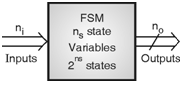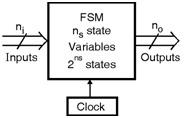Home > finite state machines > State Machine Fundamentals > Finite State Machines

# Finite State Machines

For a sequential logic system number of outputs (no) depend on the present and past, values of the inputs. Sequential logic systems are known as as finite-state machines (FSMs). FSMs are considered to have a number of internal states, which are determined by some combination of values of the ns state variablesïƒ— The FSM changes to a new state depending upon the present state and the inputs. The outputs depend on the present state and the inputs (Mealy machine) or just the present state (Moore machine).There are two types of FSMs, synchronous FSM and asynchronous FSM.

Synchronous FSM :
(The operation of a synchronous FSM is carried out by using a clock. At each clock'event the state changes to a new state which is determined by the present state and inputs.Asynchronous FSM :

Asynchronous sequential systems do not have clock and the internal states changes depending upon the change in inputs. Asynchronous FSMs are mainly used where a fast response to input changes. Asynchronous FSMs are also used where the introduction of extra frequency components related to the clock should be avoided.FSM is a type of sequential circuit which is designed to sequence through the finite states in a predetermined sequential manner. An FSM consists of three parts :
(1) Sequential current state register
(2) Combinational next state logic
(3) Combinational output logic

(1) Sequential current state register :

In this register set of n-bit flip-flops are used and are clocked by clock signal to hold the state vector of the FSM. For the state vector of n-bit 2n possible binary patterns are used for state encoding.

(2) Combinational next state logic :

As we know that, the FSM stays in a single state and at each active transition it changes from the current state to the next state. The next state is always a function of the inputs and its current state.

(3) Combinational output logic :

Outputs in FSM seems to be the function of the current state and primary inputs. Generaliy in a Moore FSM, the user wants to derive the outputs from the next state.